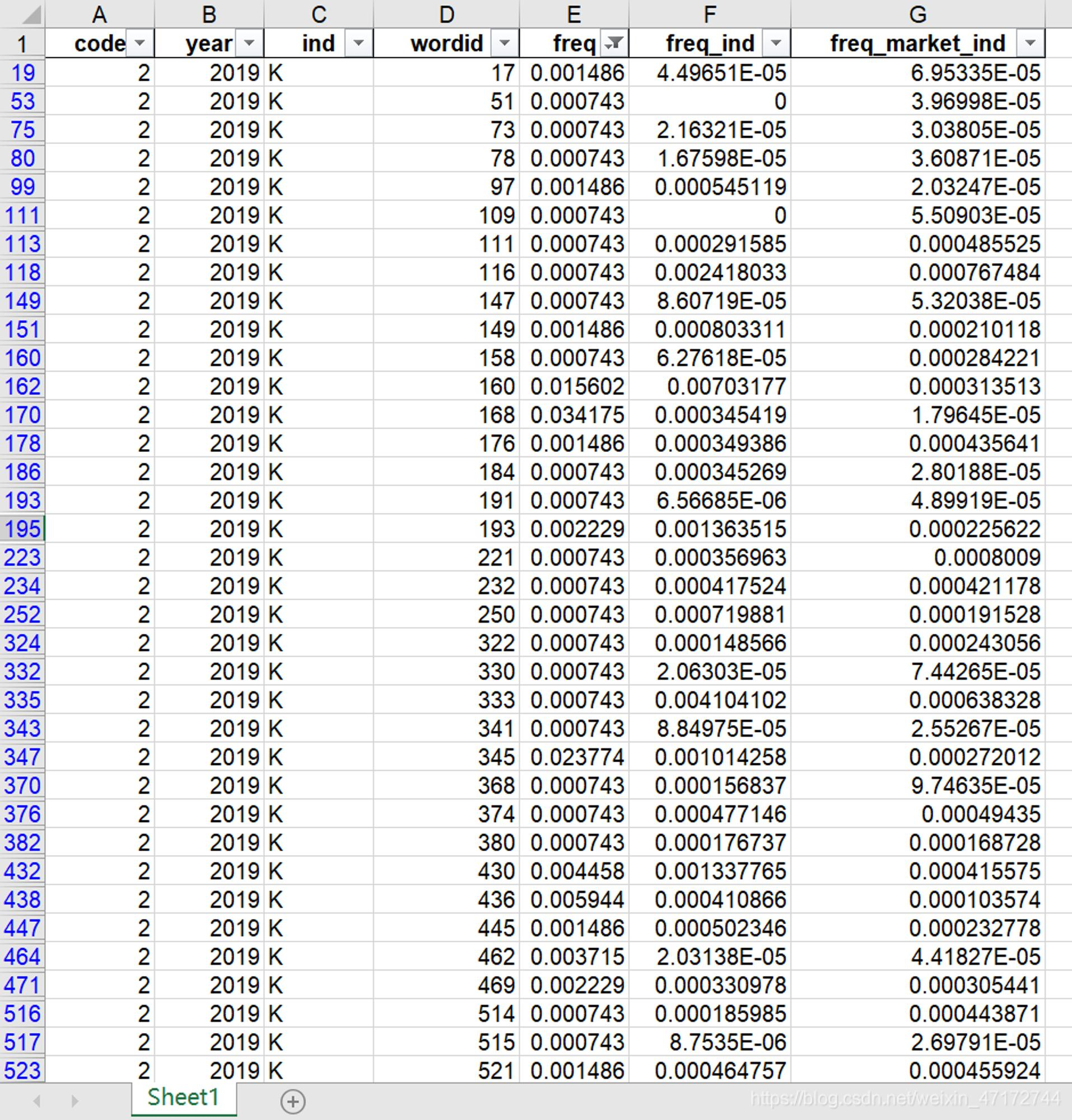墨滴Wyl

2021/04/23  阅读：18  主题：兰青

## 指标构建过程

1. 剔除金融行业、ST和*ST类企业，以及上市时间不足一年的企业

2. 从年报中提取MD&A文本内容

3. 分词处理，语料清洗

4. 文本向量化

5. 向量标准化

6. 构建回归模型

## 复刻过程

##### 数据准备
# 读取MD&A内容mda = pd.read_excel('管理层讨论与分析.xls', sheet_name = 0)# 读取行业数据industry = pd.read_excel('证监会2012年版行业分类.xlsx',sheet_name = 0)# 与行业数据进行合并data = pd.merge(mda, industry,on=['股票代码','会计年度'], how = 'inner')# 仅作剔除金融行业处理data = data[~data["industrycode2"].str.contains("J")]# 仅处理2019年的文本data = data[data["会计年度"] == 2019]# 重置索引data.reset_index(drop=True)
##### 设定分词及清理停用词函数
import jiebaimport restoplist = [i.strip() for i in open('停用词.txt', encoding = 'utf-8').readlines()]def m_cut(intxt):    word = [w for w in jieba.cut(intxt) if w not in stoplist and len(w) > 1 and not re.match('^[a-z|A-Z|0-9|.]*\$',w)]    strword = " ".join(word)    return strword
##### 对MD&A分词处理
data['cut'] = data['经营讨论与分析内容'].apply(m_cut)
##### 文本向量化

from sklearn.feature_extraction.text import CountVectorizer# 在50份以上以及在1000份MD&A出现term的才保留，看实际情况处理countvec = CountVectorizer(min_df = 50, max_df = 1000) # 生成稀疏bow矩阵res = countvec.fit_transform(data.cut) # 生成标准文档词条矩阵doc_term_matrix = res.toarray()
##### 向量标准化

import numpy as npdef normalizer(vec):    denom = np.sum(vec)    return [el / denom for el in vec]doc_term_matrix_normalizer = []for vec in doc_term_matrix:    doc_term_matrix_normalizer.append(normalizer(vec))
##### 宽数据转换为长数据，并计算行业标准化向量和市场标准化向量

for index in range(0,3568): # 2019年公司数量    df_code = df[index:index+1]    df_code = df_code.melt(id_vars=['code','year','ind'],    # 要保留的字段            var_name="wordid",   # 拉长的分类变量            value_name="freq")   # 拉长的度量值名称 # 计算行业标准化向量    dct_ind = dict(df[df["ind"] == df.iloc[index]["ind"]][df["code"] != df.iloc[index]["code"]].iloc[:,0:7308].mean(axis=0))    lt_ind = [dct_ind]    df_ind = pd.DataFrame(lt_ind)    df_ind = df_ind.melt(var_name="wordid",             value_name="freq_ind") # 计算市场标准化向量        dct_market_ind = dict(df[df["ind"] != df.iloc[index]["ind"] ].iloc[:,0:7308].mean(axis=0))    lt_market_ind = [dct_market_ind]    df_market_ind = pd.DataFrame(lt_market_ind)    df_market_ind = df_market_ind.melt(var_name="wordid",            value_name="freq_market_ind")  # 合并数据    df_code["freq_ind"] = list(df_ind["freq_ind"])    df_code["freq_market_ind"] = list(df_market_ind["freq_market_ind"])# 保存每家公司的词条文件        df_code.to_excel("F:/Rawdata/管理层讨论与分析/文档词条矩阵/{}词条.xls".format(df.iloc[index]["code"]),index=None)## 参考文献

孟庆斌,杨俊华,鲁冰.管理层讨论与分析披露的信息含量与股价崩盘风险——基于文本向量化方法的研究[J]. 中国工业经济, 2017(12): 132-150.

Hanley, K. W., and G. Hoberg. The Information Content of IPO Prospectuses[J]. Review of Financial Studies, 2010, 23(7): 2821-2864.Wyl

2021/04/23  阅读：18  主题：兰青